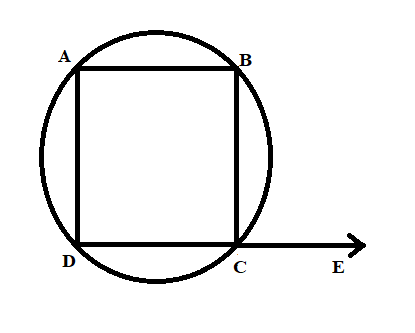QUESTION

# Prove that an exterior angle of cyclic quadrilateral is congruent to the angle opposite to its adjacent interior angle.

Hint:In this question first, we draw a figure in which a quadrilateral is inside a circle. We draw a straight line from one of the vertices of the quadrilateral and take a point on the corresponding line but the point should be exterior to the circle. We have to prove that “exterior angle of a cyclic quadrilateral is congruent to the angle opposite to its adjacent interior angle”.We are required to prove that $\angle BCE$ and $\angle BAD$ are the congruent.
Proof: The angle BCD and the angle BCE forms a linear pair. So, the sum of both the angles are ${{180}^{\circ }}$ which is evident from the figure. Therefore, we form equation (1) as:
$\angle BCE+\angle BCD={{180}^{\circ }}...(1)$
Similarly, the sum of angle BAD and the angle BCD are ${{180}^{\circ }}$ by the property that the opposite angle of cyclic quadrilateral are ${{180}^{\circ }}$.
$\angle BAD+\angle BCD={{180}^{\circ }}...(2)$
$\angle BCE\simeq \angle BAD$